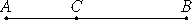# Proposition 74

If from a medial straight line there is subtracted a medial straight line which is commensurable with the whole in square only and which contains with the whole a rational rectangle, then the remainder is irrational; let it be called first apotome of a medial straight line.

From the medial straight line AB let there be subtracted the medial straight line BC which is commensurable with AB in square only and with AB makes the rectangle AB by BC rational.

I say that the remainder AC is irrational, and let it be called an apotome of a medial straight line.Since AB and BC are medial, the squares on AB and BC are also medial. But twice the rectangle AB by BC is rational, therefore the sum of the squares on AB and BC is incommensurable with twice the rectangle AB by BC.

cf. II.7
X.16

Therefore twice the rectangle AB by BC is also incommensurable with the remainder, the square on AC, since, if the whole is incommensurable with one of the magnitudes, then the original magnitudes are also incommensurable.

X.Def.I.4

But twice the rectangle AB by BC is rational, therefore the square on AC is irrational, therefore AC is irrational. Let it be called a first apotome of a medial straight line.

Q.E.D.

## Guide

This proposition is used for a few later propositions in Book X starting with X.80.